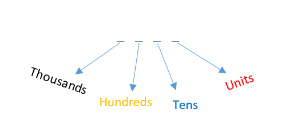QuestionAnswers

# How many different numbers of 4 digits each can be formed with the ten digits 0, 1, 2, … , 9. When digits are not repeated?Verified
92.1k+ views
Hint: A simple logic problem. We know that in the four digit number we have 4 places. If we put a zero in the thousands place it will become a three digit number. So we are not going to fill in thousands of places with zero. Also given that no digits are repeated. We find the possibilities in each place and multiply them to get the required answer.

Given, ten digits are 0, 1, 2, 3, 4, 5, 6, 7, 8, and 9.
If zero is placed in the thousand place then it becomes a three digit number out of four places.
Thus, we can fill any one of the digits except zero.
Therefore, the number of possibilities in the thousand place is = 9.
Now there are 9 digits out of given 10 digits including 0.
Again, consider the hundreds place. Here we can fill zero and any of the eight digits
Thus, the number of possibilities in hundreds places is = 9.
Now there are 8 digits out of the given 10 digits.
Considering the tenth place, we can fill any of the eight digits.
Thus the number of possibilities in tenth place is = 8.
Now there are 7 digits out of 10 digits.
Now in the unit place, here we can fill the remaining 7 digits.
Thus the number of possibilities in the unit place is = 7.
Hence, the total numbers of possibilities of a four digit number without reparation using the ten digits are
$= 9 \times 9 \times 8 \times 7$
$= 4536$ is the required answer.
So, the correct answer is “4536”.

Note: To have a clarity about the four digit number about the places, look in the below diagram.We have explained every part clearly and go through it. Just be careful about the zero digit. After finding each possibility, all we did is multiply each possibility to obtain the required answer.